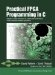# Section 13.2. Expressing the Algorithm in C

### 13.2. Expressing the Algorithm in C

As with any application destined for FPGA acceleration, the first step is to model the application at a high level, using C language. In the case of the Mandelbrot set generation algorithm, we could write the relatively simple program shown in Figure 13-2. This program generates an image (in the form of a Windows bitmap format file) representing one region of the Mandelbrot set, as defined by the following declarations:

##### Figure 13-2. Mandelbrot generation, initial floating-point version.
` #define XL -0.396562500000 #define XH -0.380470703125 #define YL  0.588052734375 #define YH  0.604144531250 // Mandelbrot generation program, floating point version. #include <windows.h> #include <sys/types.h> #include <sys/stat.h> #include <stdio.h> #include "mand.h" #define XL -0.396562500000 #define XH -0.380470703125 #define YL  0.588052734375 #define YH  0.604144531250 void mandelbrot(double xmax, double xmin, double ymax, double ymin,          double dx, double dy, double hdx, double hdy) {   int max_iterations = MAX_ITERATIONS;   double c_imag,c_real;   int j, i, t, k, R, G, B;   double result, tmp, z_real, z_imag;   // 1. Open the BMP file and write the header information   BITMAPFILEHEADER header;  /* Bitmap header */   BITMAPINFO info;          /* Bitmap information */   unsigned int pixelValue byteValue;   const char * FileName = "Mandelbrot_float.bmp";   FILE * outfile;   outfile = fopen(FileName, "wb");   if (outfile==NULL) {       fprintf(stderr, "Error opening BMP file %s for writing\n", FileName);       exit(-1);   }   header.bfType = 0x4d42; // BMP file   header.bfSize = XSIZE * YSIZE + 54;   header.bfOffBits = 54;   info.bmiHeader.biSize = 40;   info.bmiHeader.biWidth = XSIZE;   info.bmiHeader.biHeight = YSIZE;   info.bmiHeader.biPlanes = 1;   info.bmiHeader.biBitCount = 24;   info.bmiHeader.biCompression = 0;   info.bmiHeader.biSizeImage = XSIZE * YSIZE;   info.bmiHeader.biXPelsPerMeter = 11811;   info.bmiHeader.biYPelsPerMeter = 11811;   info.bmiHeader.biClrUsed = 0;   info.bmiHeader.biClrImportant = 0;   if (fwrite(&header, 1, sizeof(BITMAPFILEHEADER), outfile) < 1) {       fprintf(stderr, "Error writing BMP header for %s\n", FileName);       exit(-1);   }   if (fwrite(&info, 1, sizeof(BITMAPINFO), outfile) < 1) {       fprintf(stderr, "Error writing BMP info for %s\n", FileName);       exit(-1);   }   // 2. Calculate the value at each point   c_imag = ymax;   for (j = 0; j < YSIZE; j++) {       c_real = xmin;       for (i = 0; i < XSIZE; i++) {           z_real = z_imag = 0;           // Calculate z0, z1, .... until divergence or maximum iterations           k = 0;           do {             tmp = z_real*z_real - z_imag*z_imag + c_real;             z_imag = 2.0*z_real*z_imag + c_imag;             z_real = tmp;             result = z_real*z_real + z_imag*z_imag;             k++;           }  while (result < 4.0 && k < max_iterations);           // 3. Map points to gray scale: change to suit your preferences           B = G = R = 0;           if (k != MAX_ITERATIONS) {               R = G = G = k > 255 ? 255 : k;           }           putc(B, outfile); putc(G, outfile); putc(R, outfile);           c_real += dx;       }       c_imag -= dy;   }   fclose(outfile); } int main(int argc, char *argv[]) {     double xmin,xmax,ymin,ymax,dx,dy;     xmin = XL; xmax = XH; ymin = YL; ymax = YH;     dx = (XH-XL)/XSIZE; dy = (YH-YL)/YSIZE;     mandelbrot(xmax,xmin,ymax,ymin,dx,dy,dx/2.0,dy/2.0);     return(0); } `

These values could be passed into the program on the command line, but for simplicity we have hard-coded them as shown.

As written, the program consists of just two functions, main and mandelbrot. The main function simply calls the mandelbrot function with the predefined arguments representing the X and Y bounding values, which are assumed to be double-precision floating-point values in the range of positive 2 to negative 2. The main function also calculates scaling values for X and Y, based on the height and width (in pixels) of the desired image.

The mandelbrot function has three important sections that correspond to the following operations:

1. The output file (a BMP format file) is opened, and appropriate header information is generated. The program makes use of the Windows types BITMAPFILEHEADER and BITMAPINFO, which are further defined (for non-Windows platforms) in the file mand.h.

2. For each pixel in the desired output (which has a size of HSIZE-by-YSIZE pixels), the program calculates the value of the point. It does this by iterating on the same point until the value at that point either diverges to infinity (has a value greater than or equal to 4) or has exceeded the maximum number of iterations without yet diverging (indicating a likely convergence).

3. For each value generated in this way, the program assigns a color to the pixel based on the iteration value reached before the value diverged to infinity. For the sake of brevity, we have simply chosen a grayscale output, and have accomplished this by setting all three color values to the iteration value, up to a maximum of 255.

If you compile this program and run it, you obtain an image file very much like the image shown in Figure 13-1. This version of the application can now serve as our benchmark for the modifications to come.Practical FPGA Programming in C
ISBN: 0131543180
EAN: 2147483647
Year: 2005
Pages: 208